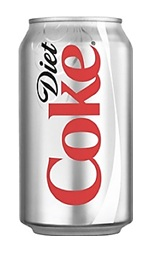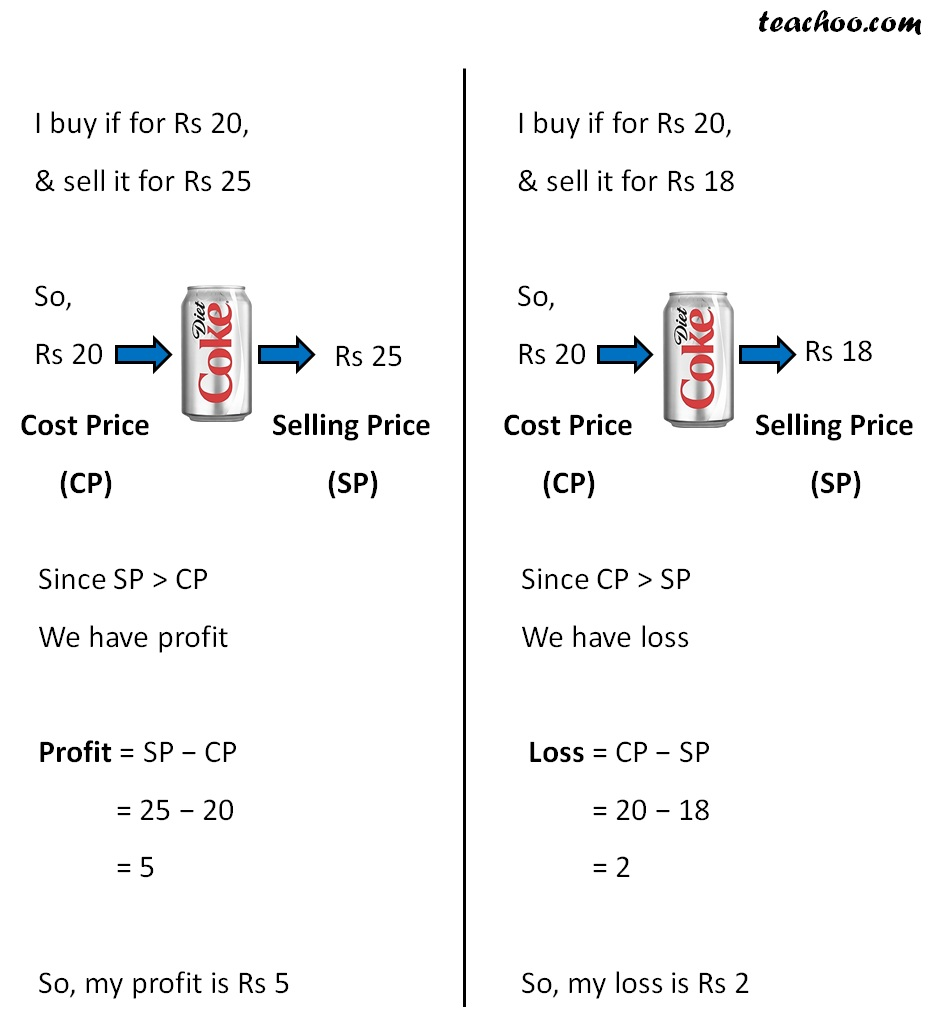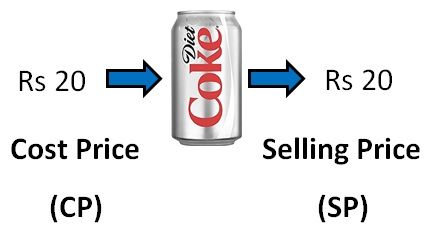Suppose I have a coke canNow,

#### Suppose I purchased the can for Rs 20 and sell it for Rs 20. What is my profit or loss?Now,

CP = Rs 20

SP = Rs 20

So,

CP = SP

Profit or Loss = CP − SP

= 20 − 20

= 0

So, when

Cost Price = Selling Price

There is no Profit, no Loss

1. Chapter 8 Class 8 Comparing Quantities
2. Concept wise
3. Finding cost price, selling price, profit % , loss%

Finding cost price, selling price, profit % , loss%